# mathman1982

•### parametric graphing

Activity

mathman1982

•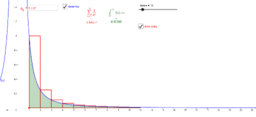### integral test

Activity

mathman1982

•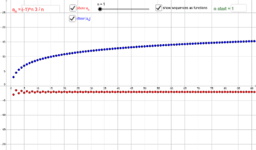### absolute convergence

Activity

mathman1982

•### Newton-Rhapson method

Activity

mathman1982

•### area in polar coordinates

Activity

mathman1982

•### graph in polar coordinates

Activity

mathman1982

•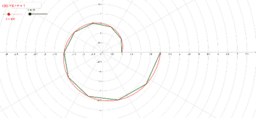### arc length in polar coordinates

Activity

mathman1982

•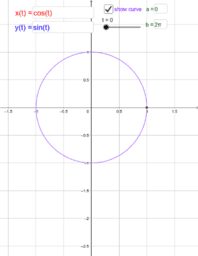### parametric equations in 2d

Activity

mathman1982

•### area between two curves in 2d

Activity

mathman1982

•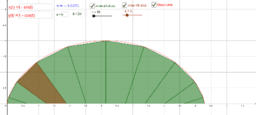### area between curve and x-axis

Activity

mathman1982

•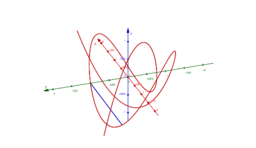### arc length in 3d

Activity

mathman1982

•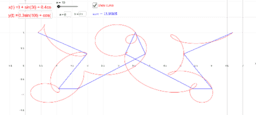### arc length in 2d

Activity

mathman1982

•### a blob from thin squares

Activity

mathman1982

•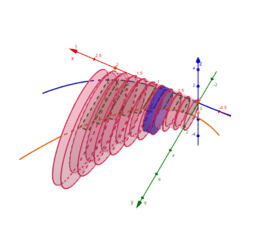### rectangles rotated about a curve and bounded by a curve

Activity

mathman1982

•### rectangles rotated about a curve

Activity

mathman1982

•### thin rectangles from the x-axis rotated about a curve

Activity

mathman1982

•### mean value theorem for integrals

Activity

mathman1982

•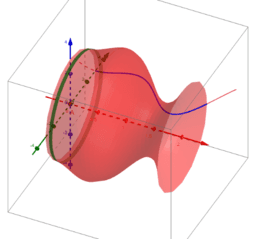### surface area of a solid of revolution

Activity

mathman1982

•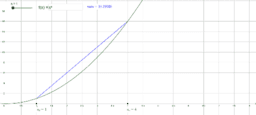### arc length

Activity

mathman1982

•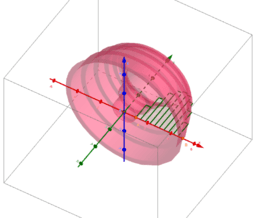### vertical rectangles rotated about the y-axis

Activity

mathman1982

•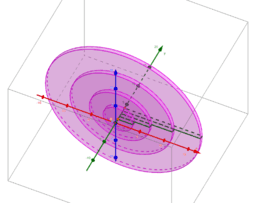### horizontal rectangles rotated about the y-axis

Activity

mathman1982

•### horizontal rectangles rotated by the x-axis

Activity

mathman1982

•### vertical rectangles rotated about the x-axis

Activity

mathman1982

•Activity

mathman1982